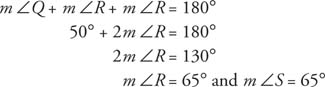## Special Features of Isosceles Triangles

Isosceles triangles are special and because of that there are unique relationships that involve their internal line segments. Consider isosceles triangle ABC  in Figure 1.Figure 1 An isosceles triangle with a median.

With a median drawn from the vertex to the base, BC , it can be proven that Δ BAX ≅ Δ CAX, which leads to several important theorems.

Theorem 32: If two sides of a triangle are equal, then the angles opposite those sides are also equal.

Theorem 33: If a triangle is equilateral, then it is also equiangular.

Theorem 34: If two angles of a triangle are equal, then the sides opposite these angles are also equal.

Theorem 35: If a triangle is equiangular, then it is also equilateral.

Example 1: Figure has Δ QRS with QR = QS. If mQ = 50°, find mR and mS.Figure 2 An isosceles triangle with a specified vertex angle.

Because mQ + mR + mS = 180°, and because QR = QS implies that mR = mS,Example 2: Figure 3 has Δ ABC with mA = mB = mC, and AB = 6. Find BC and AC.Figure 3 An equiangular triangle with a specified side.

Because the triangle is equiangular, it is also equilateral. Therefore, BC = AC = 6.